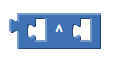# Sorting numbers and multiply each numbers

Hello,
I'm very new in MIT apps inventor.I still don't understands few blocks and how to use them.So,I have a question.Can anyone tell me how to make an instruction on how to calculate each digit of a number and can you show me have to do it .I want to make the apps to multiply each digits by a number wanted by the users and sum all of it.For example, if the user enters "2351" and multiply by "2" it will be "2×2+3×2+5×2+1×2=(4)+(6)+(10)+(2)=22".Thank you for reading this:)

You can make things easier for you if you save your multiplication until after you sum the digits,
since multiplication is distributive.

``````2*2 + 3*2 + 5*2 + 1*2 = 22 = (2+3+5+1) * 2
``````

To get a list of digits, split the number at '' (empty string) using the text split block.
You will need to remove item 1, a leftover empty string.

``````set sum = 0
for each item in the list
set sum = sum + item
(end for each)
set sum = sum * your multiplier number (2?)``````
2 Likes

Do what @ABG suggests:

1 Like

Thank you vm.I will try and ask again if I dont understand anything.

TQ i will try it first:)

Im really sorry.But I forgot to add that After the user enter the numbers(2351).I wont just multiply by "2". But it will also be powered by its index.For example "(2×2^1)+(3×2^2)+(5×2^3)+(1×2^4)" Is it possible to do it?

yes it is
use additionally a counter variable and increments its value inside the for each in list loop
the power block you can find here http://ai2.appinventor.mit.edu/reference/blocks/math.html#exponentplease try something and if you get stuck, post a screenshot of your relevant blocks

Taifun

Trying to push the limits! Snippets, Tutorials and Extensions from Pura Vida Apps byTaifun.

1 Like

Ok tq I will try adding it

1 Like

1 Like

Im sorry.I will ask carefully nest time.Thank you.

Thank you for the help1 Like

This topic was automatically closed 7 days after the last reply. New replies are no longer allowed.